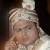# Continuous discrete varibles in Regression

Six Sigma – iSixSigma Forums Old Forums General Continuous discrete varibles in Regression

Viewing 2 posts - 1 through 2 (of 2 total)
• Author
Posts
• #31844McNiven
Member

How is best way to handle a “regression” problem when the independent variables are both continuous (eg. taxes) and discrete (eg. # of rooms) and the dependent variable is continous (eg. selling price)?  Perhaps the simplest thing to do is to convert the continuous variables to discrete ones and use a logistic regression, but I wondered what else could be done?

0
#84351Robert Butler
Participant

The simplest way to handle this is to build a regression model for taxes and then build a second one for number of rooms.  You can view your number of rooms measurement as “very granular data” and run your regression as though number of rooms was a continuous variable.  Your predicted room values will have to be rounded to the nearest integer value.
Your residual plots for rooms will look a bit odd. If your regressors do an adequate job of predicting the number of rooms you will see stratified bands of parallel data points (assuming you are plotting the residuals against the predicted variable) all crossing the zero line at the same angle.

0
Viewing 2 posts - 1 through 2 (of 2 total)

The forum ‘General’ is closed to new topics and replies.